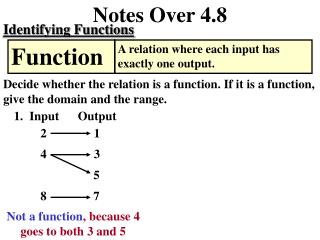DownloadDownload PresentationNotes Over 4.8

# Notes Over 4.8

Download Presentation## Notes Over 4.8

- - - - - - - - - - - - - - - - - - - - - - - - - - - E N D - - - - - - - - - - - - - - - - - - - - - - - - - - -
##### Presentation Transcript

1. Notes Over 4.8 • 1 • 3 • 5 • 8 7 Identifying Functions Function A relation where each input has exactly one output. Decide whether the relation is a function. If it is a function, give the domain and the range. 1. Input Output Not a function, because 4 goes to both 3 and 5

2. Notes Over 4.8 1 1 2 4 3 9 4 16 Identifying Functions Function A relation where each input has exactly one output. Decide whether the relation is a function. If it is a function, give the domain and the range. 2. Input Output Domain: the input Range: the output 1, 2, 3, 4 1, 4, 9, 16 A function, because every input goes to only one output

3. Notes Over 4.8 Domain: the input Range: the output 1 2 4 3 6 4 8 Identifying Functions Function A relation where each input has exactly one output. Decide whether the relation is a function. If it is a function, give the domain and the range. 3. Input Output 1, 2, 3, 4 4, 6, 8 A function, because every input goes to only one output

4. Notes Over 4.8 Identifying Functions Function Notation The equation y = 3x – 4 becomes f(x) = 3x – 4, where the solution (x, y) becomes (x, f(x)). Evaluate the function when x =3, x = 0, x = - 2.

5. Notes Over 4.8 Identifying Functions Function Notation The equation y = 3x – 4 becomes f(x) = 3x – 4, where the solution (x, y) becomes (x, f(x)). Evaluate the function when x =3, x = 0, x = - 2.

6. Notes Over 4.8 Identifying Functions Function Notation The equation y = 3x – 4 becomes f(x) = 3x – 4, where the solution (x, y) becomes (x, f(x)). Evaluate the function when x =3, x = 0, x = - 2.

7. Notes Over 4.8 Writing and Using a Linear Function 7. While on vacation, your family traveled 2040 miles in 6 days. Your average speed was miles per day. 340 a. Write a linear function that models the distance that your family traveled each day. Verbal Model Distance traveled Average speed Time Labels: Distance traveled = f ( t ) Average speed = 340 miles per day Time = t days

8. Notes Over 4.8 Writing and Using a Linear Function 7. While on vacation, your family traveled 2040 miles in 6 days. Your average speed was 340 miles per day. b. Use the model to find the distance traveled after 1.5 days of travel.

9. Notes Over 4.8 Verbal Model Distance traveled Average speed Time Labels: Distance traveled = f ( t ) Average speed = 380 miles per day Time = t days Writing and Using a Linear Function 7. While on vacation, your family traveled 2660 miles in 7 days. Your average speed was miles per day. 380 a. Write a linear function that models the distance that your family traveled each day.

10. Notes Over 4.8 Writing and Using a Linear Function 7. While on vacation, your family traveled 2660 miles in 7 days. Your average speed was 380 miles per day. b. Use the model to find the distance traveled after 1.5 days of travel.

11. Notes Over 4.8